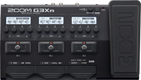# Leslie West Fat Sound

Discussion in 'Zoom G3n/G3Xn/G5n' started by gianni, Apr 28, 2019.

1. Leslie West Fat SoundDevice: Zoom G3Xn
Firmware: 2.10

Name on device: Leslie 2
Optimized for: Guitar Amp

Effects chain:Leslie West Sound

Effect: "ZNR" (Dynamics), active - "yes"
"Detect" = EFXIN
"Depth" = 75
"Threshold" = 33
"Decay" = 12

Effect: "OutputBST" (Overdrive / Distortion), active - "yes"
"Range" = 4
"Boost" = 40
"Tone" = 50
"Volume" = 90

Effect: "MetalWRLD" (Overdrive / Distortion), active - "yes"
"Gain" = 71
"Bass" = 42
"Treble" = 31
"Volume" = 100

Effect: "Gt GEQ" (Filter), active - "yes"
"160 Hz" = -3.0
"400 Hz" = -4.5
"800 Hz" = -4.5
"3.2 kHz" = -6.5
"6.4 kHz" = 2.5
"12.0 kHz" = 2.5
"Volume" = 95
"CH SEL" = UnLATCH
"160 Hz" = 0.0
"400 Hz" = 0.0
"800 Hz" = 0.0
"3.2 kHz" = 0.0
"6.4 kHz" = 0.0
"12.0 kHz" = 12.0
"Volume" = 1
"CH SEL" = LATCH
"Parameter set" = A

Effect: "Output VP" (Pedal), active - "yes"

Effect: "Delay" (Delay), active - "yes"
"Time" = 304
"F.B." = 51
"Mix" = 48
"Tail" = On

Patch Volume: 89